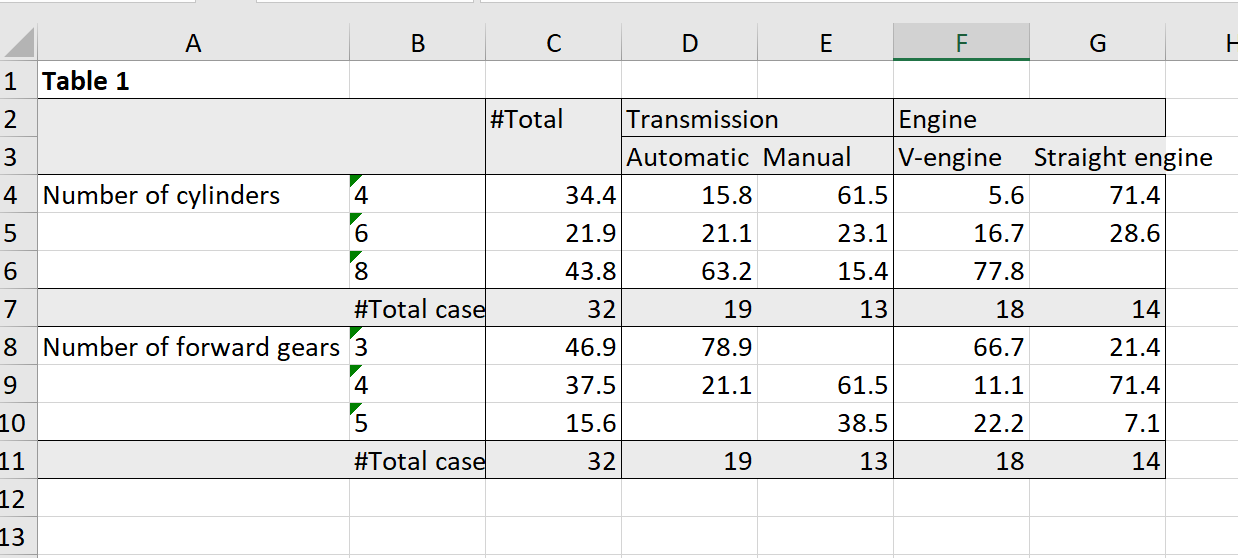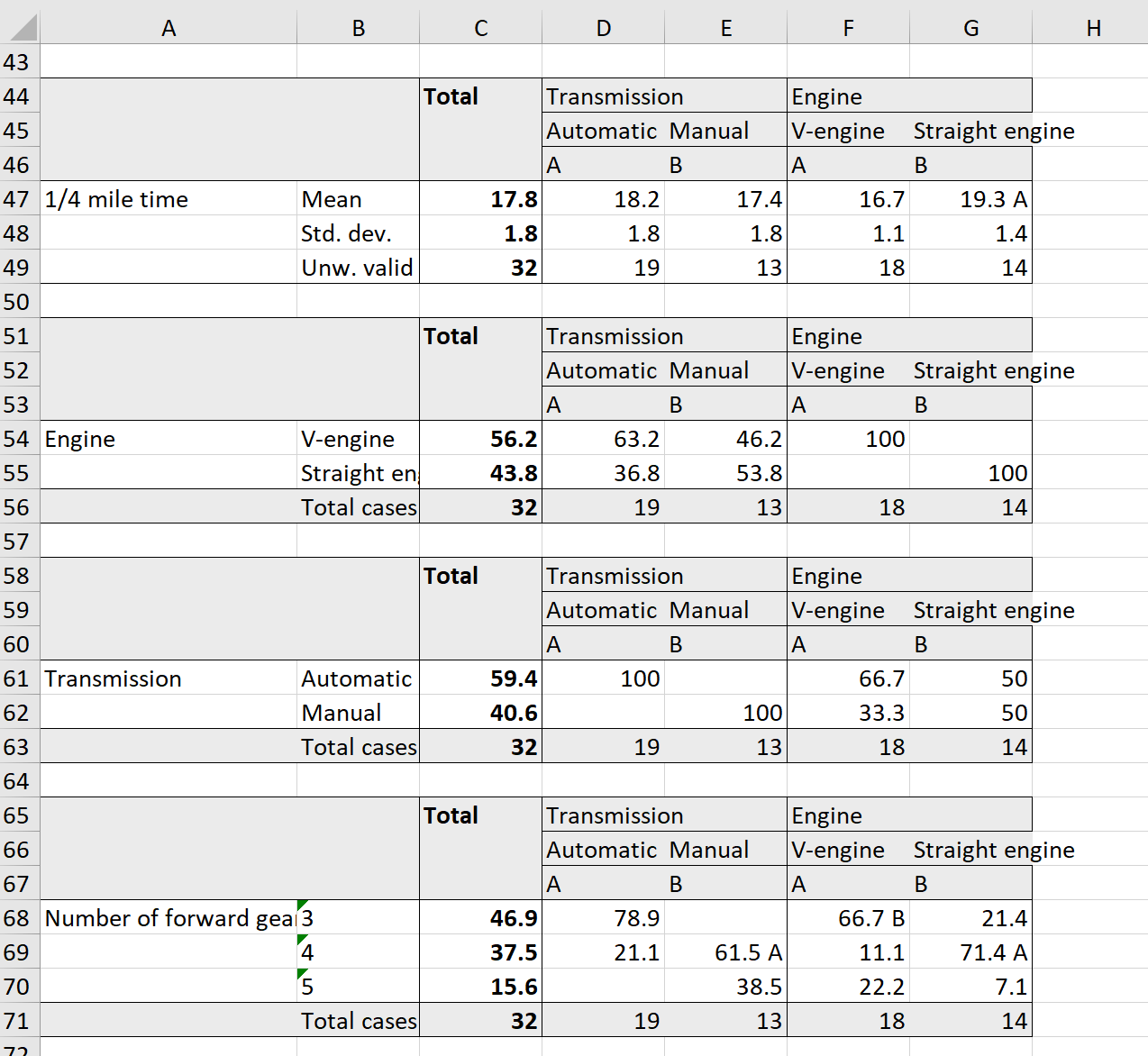## Introduction

To export expss tables to *.xlsx you need to install excellent openxlsx package. To install it just type in the console install.packages("openxlsx").

## Examples

First we apply labels on the mtcars dataset and build simple table with caption.

library(expss)
library(openxlsx)
data(mtcars)
mtcars = apply_labels(mtcars,
mpg = "Miles/(US) gallon",
cyl = "Number of cylinders",
disp = "Displacement (cu.in.)",
hp = "Gross horsepower",
drat = "Rear axle ratio",
wt = "Weight (lb/1000)",
qsec = "1/4 mile time",
vs = "Engine",
vs = c("V-engine" = 0,
"Straight engine" = 1),
am = "Transmission",
am = c("Automatic" = 0,
"Manual"=1),
gear = "Number of forward gears",
carb = "Number of carburetors"
)

mtcars_table = mtcars %>%
cross_cpct(
cell_vars = list(cyl, gear),
col_vars = list(total(), am, vs)
) %>%
set_caption("Table 1")

mtcars_table
  #Total     Transmission     Engine Table 1 Number of cylinders 4 34.4 15.8 61.5 5.6 71.4 6 21.9 21.1 23.1 16.7 28.6 8 43.8 63.2 15.4 77.8 #Total cases 32 19 13 18 14 Number of forward gears 3 46.9 78.9 66.7 21.4 4 37.5 21.1 61.5 11.1 71.4 5 15.6 38.5 22.2 7.1 #Total cases 32 19 13 18 14

Then we create workbook and add worksheet to it.

wb = createWorkbook()
sh = addWorksheet(wb, "Tables")

Export - we should specify workbook and worksheet.

xl_write(mtcars_table, wb, sh)

And, finally, we save workbook with table to the xlsx file.

saveWorkbook(wb, "table1.xlsx", overwrite = TRUE)

Screenshot of the exported table:## Automation of the report generation

First of all, we create banner which we will use for all our tables.

banner = with(mtcars, list(total(), am, vs))

Then we generate list with all tables. If variables have small number of discrete values we create column percent table. In other cases we calculate table with means. For both types of tables we mark significant differencies between groups.

list_of_tables = lapply(mtcars, function(variable) {
if(length(unique(variable))<7){
cro_cpct(variable, banner) %>% significance_cpct()
} else {
# if number of unique values greater than seven we calculate mean
cro_mean_sd_n(variable, banner) %>% significance_means()

}

})

Create workbook:

wb = createWorkbook()
sh = addWorksheet(wb, "Tables")

Here we export our list with tables with additional formatting. We remove ‘#’ sign from totals and mark total column with bold. You can read about formatting options in the manual fro xl_write (?xl_write in the console).

xl_write(list_of_tables, wb, sh,
# remove '#' sign from totals
col_symbols_to_remove = "#",
row_symbols_to_remove = "#",
# format total column as bold
other_col_labels_formats = list("#" = createStyle(textDecoration = "bold")),
other_cols_formats = list("#" = createStyle(textDecoration = "bold")),
)

Save workbook:

saveWorkbook(wb, "report.xlsx", overwrite = TRUE)

Screenshot of the generated report: